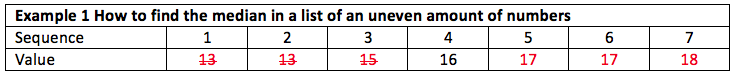# Research-Coaches.com

## Median

### The median is the value of the number in the centre of an ordered list of numbers.

If you have a long list of numbers, you would like to describe it briefly. You can compute the mean for pointing to the centre of that list of numbers. Another indicator for the centre of the list is the median.

To find the median in a list of numbers, the list has to be ordered. The best way to do this is from lowest to highest. Next you stripe away the lowest and the highest number. (Be sure you take the number and not the value of that number.) Keep doing this till only one number is left (if you have an uneven amount of numbers), or two (if you have an even amount of numbers). For instance:If you have an uneven amount of numbers only one number is left over. In an even amount of numbers it are always two. If you are lucky these values are the same, so you can pinpoint this value as the median. If you have bad luck these numbers are different. Now there are two situations:

1) There are only two numbers and they are unequal to the numbers before and after these two. Now the median is computed as the middle of these two numbers.
2) A list of equal numbers appear before or after the two that were left over. Now the median is computed as the mean of all these equal numbers.

In our example the median for the uneven amount of numbers is 16, and for the list with the even amount of numbers the median is 16.666.

## How to interpret the median

The median and mean of a list of numbers must be fairly equal to determine the center of that list. That is not always the case and if so, this is caused by outliers. If you have outliers, you should check your data because outliers have a major impact on statistical test calculations.

## Related topics to median:## The most important aspect of any researcharrow_drop_up arrow_drop_down